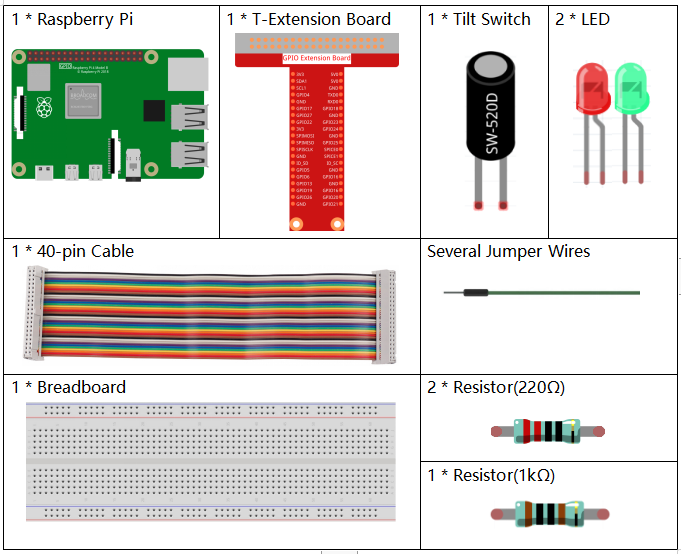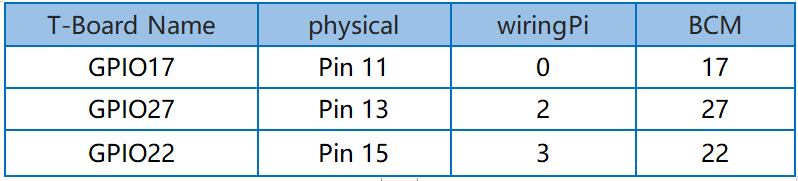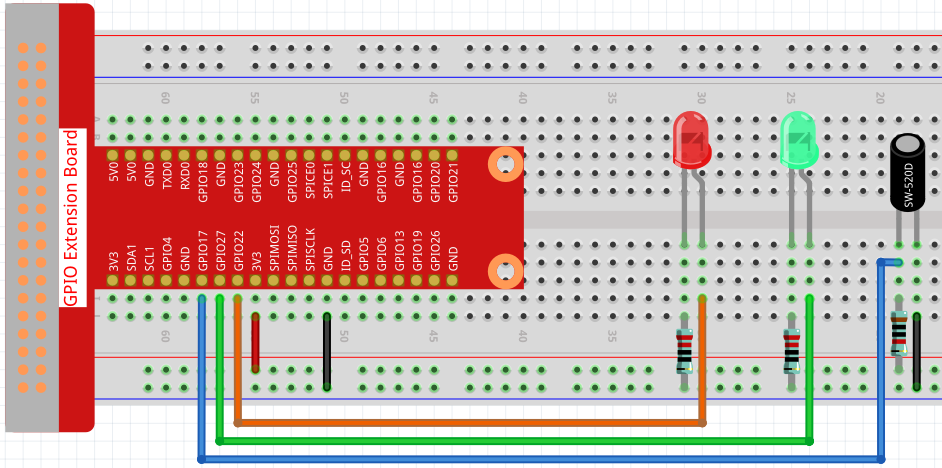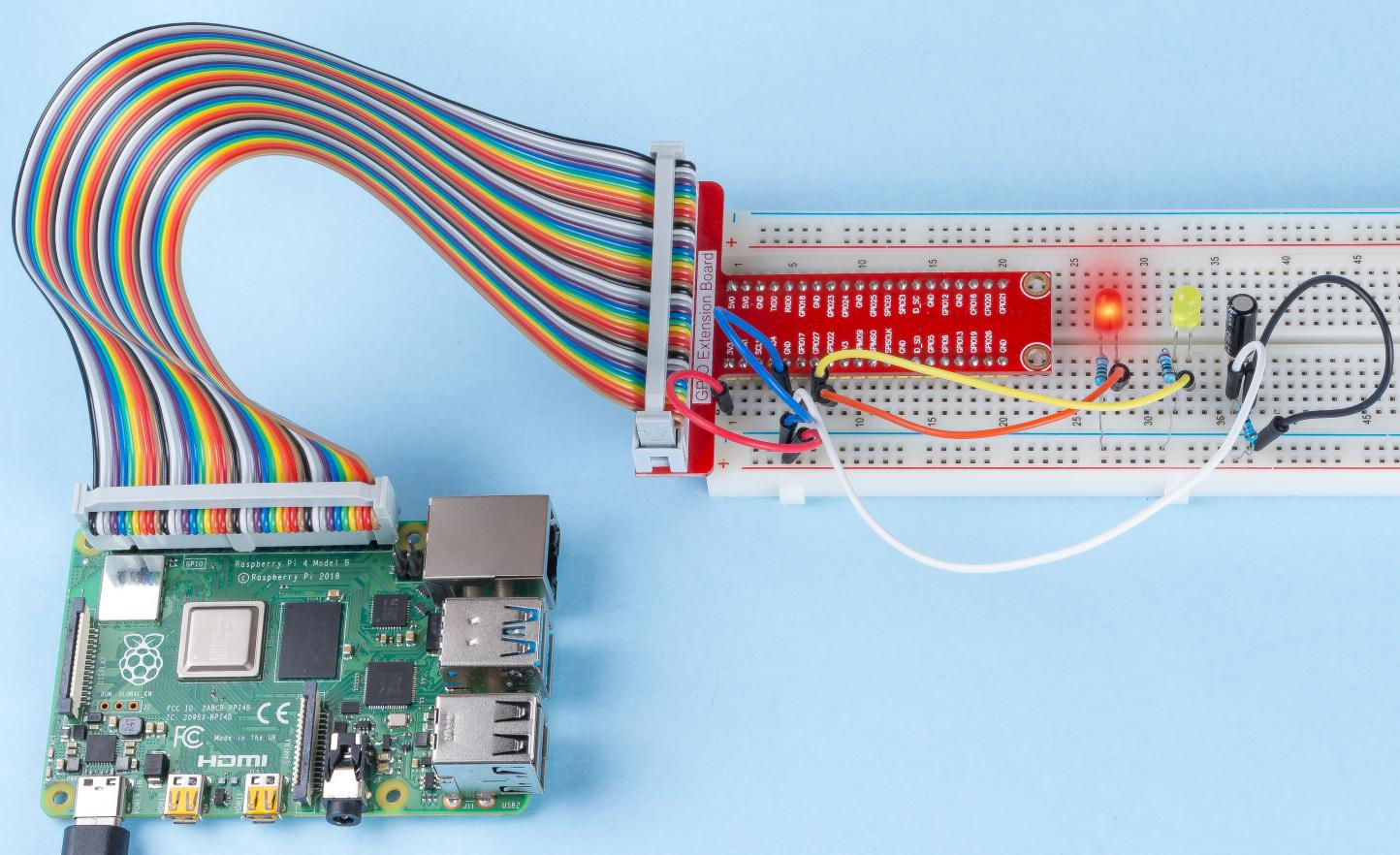# 2.1.5 Tilt Switch¶

## Introduction¶

This is a ball tilt-switch with a metal ball inside. It is used to detect inclinations of a small angle.

## Components¶## Schematic Diagram¶## Experimental Procedures¶

Step 1: Build the circuit.Step 2: Go to the folder of the code.

```cd /home/pi/raphael-kit/nodejs/
```

Step 3: Run the code.

```sudo node tilt_switch.js
```

Place the tilt vertically, and the green LED will turns on. If you tilt it, the red LED will turns on. Place it vertically again, and the green LED will lights on.

Code

```const Gpio = require('pigpio').Gpio;

const led1 = new Gpio(22, { mode: Gpio.OUTPUT });
const led2 = new Gpio(27, { mode: Gpio.OUTPUT });

const tilt = new Gpio(17, {
mode: Gpio.INPUT,
pullUpDown: Gpio.PUD_DOWN,
edge: Gpio.EITHER_EDGE
});

tilt.on('interrupt', (level) => {
if (level) {
console.log("Horizontally");
}
else {
console.log("Vertically");
}
led1.digitalWrite(level);
led2.digitalWrite(!level);
});
```

Code Explanation

```const Gpio = require('pigpio').Gpio;

const led1 = new Gpio(22, { mode: Gpio.OUTPUT });
const led2 = new Gpio(27, { mode: Gpio.OUTPUT });

const tilt = new Gpio(17, {
mode: Gpio.INPUT,
pullUpDown: Gpio.PUD_DOWN,
edge: Gpio.EITHER_EDGE
});
```

Import the pigpio module and create three objects led1, led2, tilt, By reading the level of the tilt IO port, the on and off of led1 and led2 are controlled.

```const tilt = new Gpio(17, {
mode: Gpio.INPUT,
pullUpDown: Gpio.PUD_DOWN,
edge: Gpio.EITHER_EDGE
});
```

Create a tilt object to control the IO port Gpio17, set it to input mode, pull-down resistor (initially low level). And set the interrupt function, the mode is EITHER_EDGE, that is, both rising and falling edges will trigger the interrupt function.

```tilt.on('interrupt', (level) => {
if (level) {
console.log("Horizontally");
}
else {
console.log("Vertically");
}
led1.digitalWrite(level);
led2.digitalWrite(!level);
});
```

When the interrupt is triggered, write the same level to led1, and write the opposite level to led2. When the tilt IO port is high, the terminal prints “Horizontally”; When the tilt IO port is low, the terminal prints “Vertically”.

## Phenomenon Picture¶